# How to Reverse a String in C

## How to Reverse a String in C Language with Examples

In this article, I will discuss How to Reverse a String in C Language with Examples. Please read our previous article, discussing How to Validate a String in C Language with Examples.

##### How to Reverse a String in C Language?

Reversing a string in C involves swapping characters from start to end until the middle is reached. This can be done using a temporary variable. Now, we will see how to reverse a String. Please observe the following string: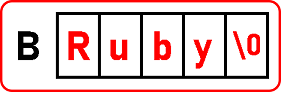We have taken a string Ruby. We want to reverse this string. The reverse string will be: ‘ybuR’. For reversing, there is more than one method.

In 1st method, we will take another array and copy the reverse form of that original string in this array. So, the original will remain the same, and we will get the reverse version of that string in another array. We have to add ‘\0’ at the end of another array. So, it becomes a string, so let us see how we can reverse it. For reversing, we have to reach the end of a string, which is the last alphabet: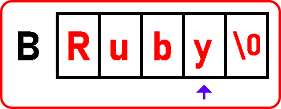So once we are on the last alphabet, we can start copying those elements in another array in reverse order as: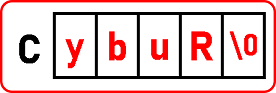So, it is just like reverse copying a string. We will take ‘j’ as the index pointer and move this until we reach ‘\0’.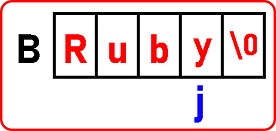So, ‘j’ is pointing to the last element. We have to copy that element into another array: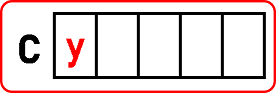We have to decrement j to the value of -1. And if j is on -1, then we have to stop there. This way, we will copy all the alphabets from the original array to our extra array in reversing order. And when we copy all the alphabets or point on ‘\0’.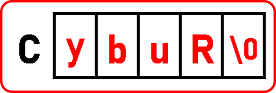Now, this becomes a string. It was the array of characters, but as we added ‘\0’, it became a string. At last, we have to print this reverse form of string. Below is the code for the above procedure:

##### Program for Reversing a String using Extra Array in C language:
```#include <stdio.h>
#include <stdlib.h>
int main ()
{
char B[] = "Ruby";
int C;
int i, j;
for (i = 0; B[i] != '\0'; i++) {
// no code here
}

i = i - 1;
for (j = 0; i >= 0; i--, j++) {
C[j] = B[i];
}

C[j] = '\0';
printf ("Original String is \"%s\"\n", B);
printf ("Original String is \"");

for (int k = 0; k < 4; k++) {
printf ("%c", C[k]);
}
printf ("\"");
return 0;
}```
###### Output: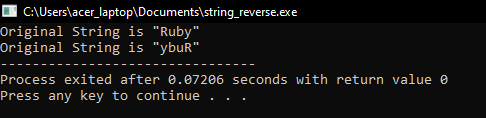##### Reverse a String without using Extra Array in C Language:

Now, let us look at the 2nd method for reversing a string. In this method, we don’t require any extra array. Those strings cannot be modified if we take a pointer instead of an array. So, make sure that you are taking a mutable string. So, we will modify the same string. Now, let us come to the procedure. Please observe the following string.We will swap the character with a suitable position, like The 4th letter with the 1st letter and the 2nd letter with the 3rd letter. In this way, we will exchange the characters of a string.

##### Program to Reverse a String without extra Array in C Language:
```#include <stdio.h>
#include <stdlib.h>
int main ()
{
char B[] = "Ruby";
char t;
int i, j;
printf ("Original String is \"%s\"\n", B);

for (j = 0; B[j] != '\0'; j++) {
// no code here
}

j = j - 1;
for (i = 0; i < j; i++, j--) {
t = B[i];
B[i] = B[j];
B[j] = t;
}

printf ("Reversed String is \"");
for (int k = 0; k < 4; k++) {
printf ("%c", B[k]);
}
printf ("\"");
return 0;
}```
###### Output:So, this is the method for reversing a string without using another array.

##### Another Approach to Reverse a String in C:

This method is a common and efficient way to reverse a string in C, as it only requires a single pass through half of the string. You will need stdio.h for input/output operations and string.h for the strlen() function.

```#include <stdio.h>
#include <string.h>

void reverseString(char *str) {
int length = strlen(str);
for (int i = 0; i < length / 2; i++) {
char temp = str[i];
str[i] = str[length - 1 - i];
str[length - 1 - i] = temp;
}
}

int main() {
char B[] = "Ruby";
printf ("Original String is \"%s\"\n", B);
reverseString(B);
printf("Reversed String: %s\n", B);
return 0;
}```
##### In this Example:
• The reverseString() function reverses the string in place, meaning it modifies the original string. Ensure that the string is modifiable (i.e., not a string literal).
• The function calculates the length of the string first, then swaps each character from the beginning with the corresponding character from the end, moving towards the center.
• This implementation only works with proper C strings (null-terminated character arrays). Ensure that your string is null-terminated.
##### Another Approach to Reverse a String in C:

Here’s an example program that demonstrates how to reverse a string in place:

```#include <stdio.h>
#include <string.h>

void reverseString(char *str) {
int left = 0;
int right = strlen(str) - 1;
char temp;

while (left < right) {
// Swap characters
temp = str[left];
str[left] = str[right];
str[right] = temp;

// Move towards middle
left++;
right--;
}
}

int main() {
char str[] = "Ruby";
reverseString(str);

printf("Reversed String: %s\n", str);

return 0;
}
```

In this program:

• reverseString is a function that takes a string (char *str) and reverses it.
• It uses two indices, left and right, to traverse the string from both ends.
• Characters at left and right indices are swapped until the middle of the string is reached.
• strlen(str) – 1 is used to get the index of the last character in the string (excluding the null terminator).
• In the main function, the string str is reversed using reverseString and then printed out.